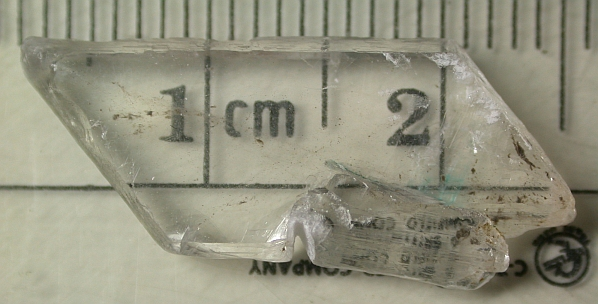Estimated optical constants of Gypsum in the regions of weak absorptions:Application of scattering theories and comparisons to independent measurements

Ted L. Roush
NASA Ames Research Center, Astrobiology & Space Sciences Division
Planetary Systems Branch, MS 245-3, Moffett Field, CA  94035-1000

Francesca Esposito
INAF, Osservatorio Astronomico di Capodimonte, Via Moiariello 16, 80131, Napoli, Italy

George R. Rossman
Division of Geological and Planetary Sciences
Califorinia Institute of Technology, Pasadena, CA  91125-2500

Luigi Colangeli
INAF, Osservatorio Astronomico di Capodimonte, Via Moiariello 16, 80131, Napoli, Italy

Abstract

Diffuse reflectance spectra of multiple grain size fractions are used to estimate the optical constants of gypsum over the 0.4-15 mm wavelength region.  Two independent scattering theories are used to iteratively determine the imaginary index of refraction from the measured reflectance.  We compare the results of these two with each other, and with imaginary indices of gypsum reported in the literature.  We find that the scattering theory results are more sensitive in the infrared to weak spectral features that are clearly distinguished in the diffuse reflectance spectra.  However, we find the scattering results provide a poor determination of the optical constants in the regions of relatively strong absorptions.  At visible and near-infrared wavelengths we provide a comparison to the results obtained from analysis of the diffuse reflectance to results obtained from direct transmission measurements of gypsum crystals.  We find the imaginary index of refraction determined from scattering theories is systematically less than that obtained from transmission spectra, especially for regions of weak absorption.  We attribute this discrepancy to potential scattering that occurs within the crystals during the transmission measurements.  We combine the resulting real and imaginary indices of refraction with those reported at infrared wavelengths to provide values covering visual, near-, and infrared wavelengths (0.4-333 mm, 25000-30 cm-1).Gypsum crystal 2.856 mm thick from Brookville, Saline County, Kansas

Data from the Paper

Figure 1, Imaginary Indices of Refraction                      Click to Save
 Aronson et al.  E \\b Long et al.  E \\a=X Long et al.  E \\b=Y Long et al.  E \\c=Z Long et al.  Average This study Pellet Marzo et al.  KBr pellet Long et al.  Pellet

Figure 2, Diffuse Reflectance Spectra
 RELAB <45 µm RELAB 25 -75 µm ASTER Fine ASTER Medium ASTER Coarse

Figure 3, Specular Reflectance of Gypsum
 This Study Pressed Pellet

Figure 4, Transmission Spectra of Four Gypsum Crystals
 Gypsum 034 E \\X Gypsum 034 E \\Z Gypsum 1171 E \\X Gypsum 1171 E \\Z Gypsum 2856 E \\X Gypsum 2856 E \\Z Gypsum 14293 E \\X Gypsum 14293 E \\Z

Figure 5, Contour Plot
 Shaded Countour Plot Data

Figure 6, Calculated Single Scattering Albedo Values
 Albedo Values Data

Figure 7, Comparison of k-values
 Hapke  ASTER data Data Shkuratov et al.  ASTER data Data Shkuratov et al.  RELAB data Data Long et al  average values Data

Figure 8, k-values
 Shkuratov et al.  ASTER Data Shkuratov et al.  RELAB Data Long et al.  average Data

Figure 9, Transmission Spectra of (010) face
 full model all Data modified model all Data simplified model all Data

Figure 10, Optical Constants of Gypsum Samples
 0.0341 cm, E \\X = ~alpha Data a 0.0341 cm, E \\Z = ~ gamma Data b 0.1171 cm, E \\X = ~alpha Data c 0.1171 cm, E \\Z = ~ gamma Data d 0.2856 cm, E \\X = ~alpha Data e 0.2856 cm, E \\Z = ~ gamma Data f 1.1493 cm, E \\X = ~alpha Data g 1.1493 cm, E \\Z = ~ gamma Data h Derived imaginary index, E \\X = ~alpha see files a-h Derived imaginary index, E \\Z = ~ gamma see files a-h

Figure 11, Derived Imaginary Indices
 0.0341 cm, E \\X = ~alpha Data 0.0341 cm, E \\Z = ~ gamma Data 0.1171 cm, E \\X = ~alpha Data 0.1171cm, E \\Z = ~ gamma Data 0.2856 cm, E \\X = ~alpha Data 0.2856 cm, E \\Z = ~ gamma Data 1.1439 cm, E \\X = ~alpha Data 1.1493 cm, E \\Z = ~ gamma Data Combined imaginary index, E \\X = ~alpha Data Combined imaginary index, E \\Z = ~ gamma Data

Figure 12, Theoretical values
 n-values Infrared Data n-values Vis-NIR Data k-values Infrared Data k-values VIS-NIR Data

Figure 13, Average n-values
 Hapke theory Data Shkuratov theory Data

Figure 14, Average n-values
 Average of all theory Data

Figure 15,  Data Comparisons
 Imaginary Index, E\\X Data Imaginary Index, E\\Z Data Transmission average Data Hapke-Shkuratov Data Sub-sampling Transmission Data Sub-sampling Hapke-Shkuratov Data

Journal of Geophysical Research 112